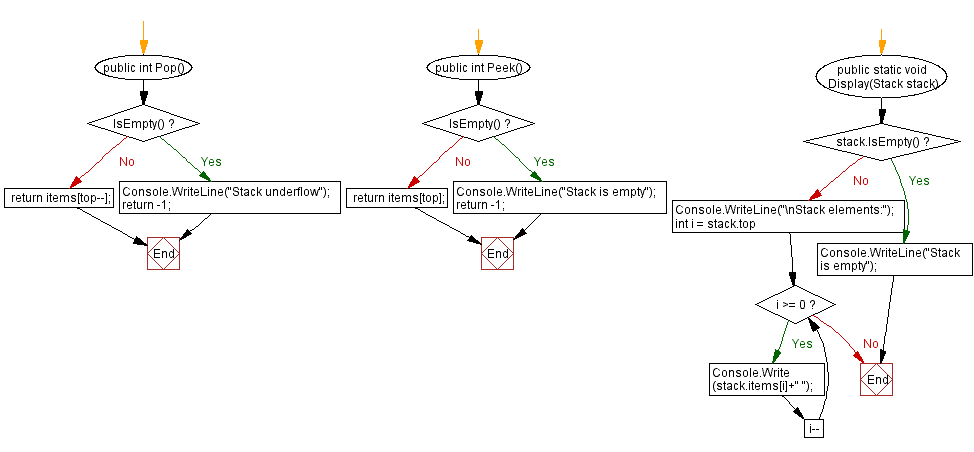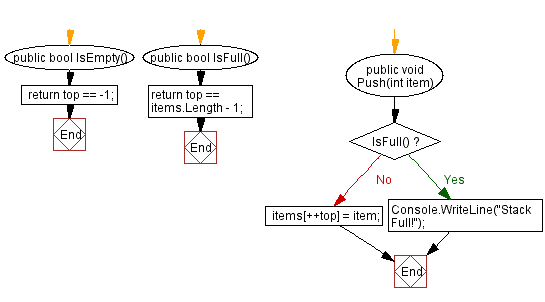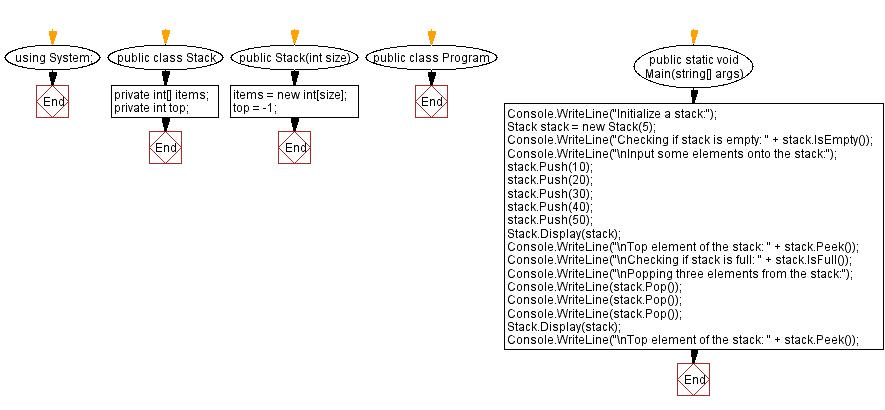﻿ C# - Implement a stack with push and pop operations

# C# Sharp Stack Exercises: Implement a stack with push and pop operations

## C# Sharp Stack: Exercise-1 with Solution

Write a C# program to implement a stack with push and pop operations. Find the top element of the stack and check if the stack is empty or not.

Sample Solution:

C# Code:

``````using System;
public class Stack
{
private int[] items;
private int top;

public Stack(int size)
{
items = new int[size];
top = -1;
}

public bool IsEmpty()
{
}

public bool IsFull()
{
}

public void Push(int item)
{
if (IsFull())
{
Console.WriteLine("Stack Full!");
return;
}

items[++top] = item;
}

public int Pop()
{
if (IsEmpty())
{
Console.WriteLine("Stack underflow");
return -1;
}

return items[top--];
}

public int Peek()
{
if (IsEmpty())
{
Console.WriteLine("Stack is empty");
return -1;
}

return items[top];
}

public static void Display(Stack stack)
{
if (stack.IsEmpty())
{
Console.WriteLine("Stack is empty");
return;
}

Console.WriteLine("\nStack elements:");
for (int i = stack.top; i >= 0; i--)
{
Console.Write(stack.items[i]+" ");
}
}

}

public class Program
{
public static void Main(string[] args)
{
Console.WriteLine("Initialize a stack:");
Stack stack = new Stack(5);
Console.WriteLine("Checking if stack is empty: " + stack.IsEmpty());
Console.WriteLine("\nInput some elements onto the stack:");
stack.Push(10);
stack.Push(20);
stack.Push(30);
stack.Push(40);
stack.Push(50);
Stack.Display(stack);
Console.WriteLine("\nTop element of the stack: " + stack.Peek());
Console.WriteLine("\nChecking if stack is full: " + stack.IsFull());
Console.WriteLine("\nPopping three elements from the stack:");
Console.WriteLine(stack.Pop());
Console.WriteLine(stack.Pop());
Console.WriteLine(stack.Pop());
Stack.Display(stack);
Console.WriteLine("\nTop element of the stack: " + stack.Peek());
}
}
``````

Sample Output:

```Initialize a stack:
Checking if stack is empty: True

Input some elements onto the stack:

Stack elements:
50 40 30 20 10
Top element of the stack: 50

Checking if stack is full: True

Popping three elements from the stack:
50
40
30

Stack elements:
20 10
Top element of the stack: 20
```

Flowchart:C# Sharp Code Editor:

Improve this sample solution and post your code through Disqus

What is the difficulty level of this exercise?

Test your Programming skills with w3resource's quiz.

﻿